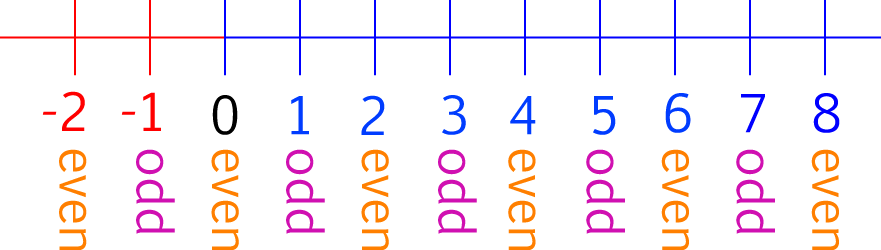# Definition of Odd NumberAn odd number is an integer that cannot be written as $2$ times another integer.

For example, $11$ is an odd number. The picture shows $11$ shapes divided up into groups of two. There is one left over. This tells us that $11$ is odd.

Every odd number has $1,3,5,7$ or $9$ as its final digit.

Some examples of odd numbers are $-579$, $-5$, $3$, $23$ and $1,542,692,377$.

### Description

The aim of this dictionary is to provide definitions to common mathematical terms. Students learn a new math skill every week at school, sometimes just before they start a new skill, if they want to look at what a specific term means, this is where this dictionary will become handy and a go-to guide for a student.

### Audience

Year 1 to Year 12 students

### Learning Objectives

Learn common math terms starting with letter O

Author: Subject Coach
You must be logged in as Student to ask a Question.